# 深入浅出负载均衡vivo互联网技术## 一、负载均衡简介

### 1.1. 大型网站面临的挑战

• 应用集群：将同一应用部署到多台机器上，组成处理集群，接收负载均衡设备分发的请求，进行处理，并返回相应数据。

• 负载均衡：将用户访问请求，通过某种算法，分发到集群中的节点。

## 二、负载均衡的分类

### 2.1 载体维度分类

#### 2.1.1 硬件负载均衡

• 功能强大：支持全局负载均衡并提供较全面的、复杂的负载均衡算法。

• 性能强悍：硬件负载均衡由于是在专用处理器上运行，因此吞吐量大，可支持单机百万以上的并发。

• 安全性高：往往具备防火墙，防 DDos 攻击等安全功能。

• 成本昂贵：购买和维护硬件负载均衡的成本都很高。

• 扩展性差：当访问量突增时，超过限度不能动态扩容。

#### 2.1.2 软件负载均衡

• LVS 可以作为四层负载均衡器。其负载均衡的性能要优于 Nginx。

• HAProxy 可以作为 HTTP 和 TCP 负载均衡器。

• Nginx、HAProxy 可以作为四层或七层负载均衡器。

• 扩展性好：适应动态变化，可以通过添加软件负载均衡实例，动态扩展到超出初始容量的能力。

• 成本低廉：软件负载均衡可以在任何标准物理设备上运行，降低了购买和运维的成本。

• 性能略差：相比于硬件负载均衡，软件负载均衡的性能要略低一些。

### 2.2 网络通信分类

1) 七层负载均衡：就是可以根据访问用户的 HTTP 请求头、URL 信息将请求转发到特定的主机。

• DNS 重定向

• HTTP 重定向

• 反向代理

2) 四层负载均衡：基于 IP 地址和端口进行请求的转发。

• 修改 IP 地址

• 修改 MAC 地址

#### 2.2.1 DNS 负载均衡

DNS 负载均衡一般用于互联网公司，复杂的业务系统不适合使用。大型网站一般使用 DNS 负载均衡作为 第一级负载均衡手段，然后在内部使用其它方式做第二级负载均衡。DNS 负载均衡属于七层负载均衡。

DNS 即 域名解析服务，是 OSI 第七层网络协议。DNS 被设计为一个树形结构的分布式应用，自上而下依次为：根域名服务器，一级域名服务器，二级域名服务器，... ，本地域名服务器。显然，如果所有数据都存储在根域名服务器，那么 DNS 查询的负载和开销会非常庞大。

DNS 负载均衡的工作原理就是：基于 DNS 查询缓存，按照负载情况返回不同服务器的 IP 地址。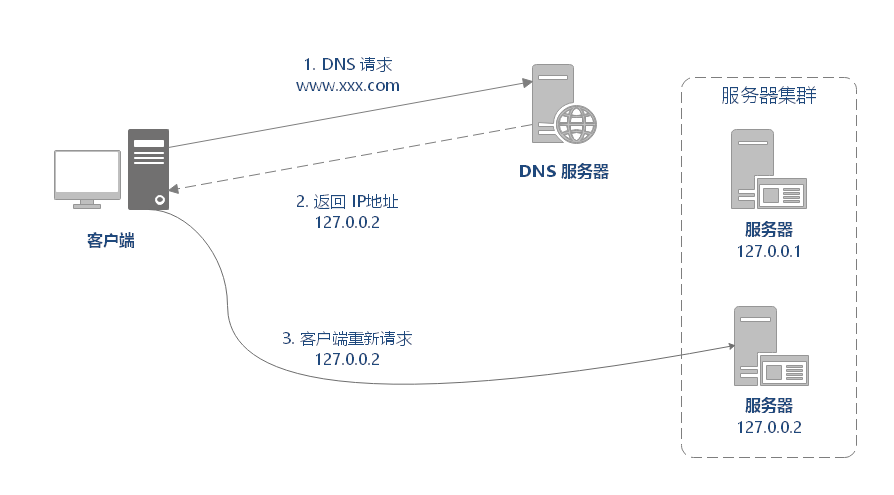DNS 重定向的 优点：

DNS 重定向的 缺点：

#### 2.2.2 HTTP 负载均衡

HTTP 负载均衡是基于 HTTP 重定向实现的。HTTP 负载均衡属于七层负载均衡。

HTTP 重定向原理是：根据用户的 HTTP 请求计算出一个真实的服务器地址，将该服务器地址写入 HTTP 重定向响应中，返回给浏览器，由浏览器重新进行访问。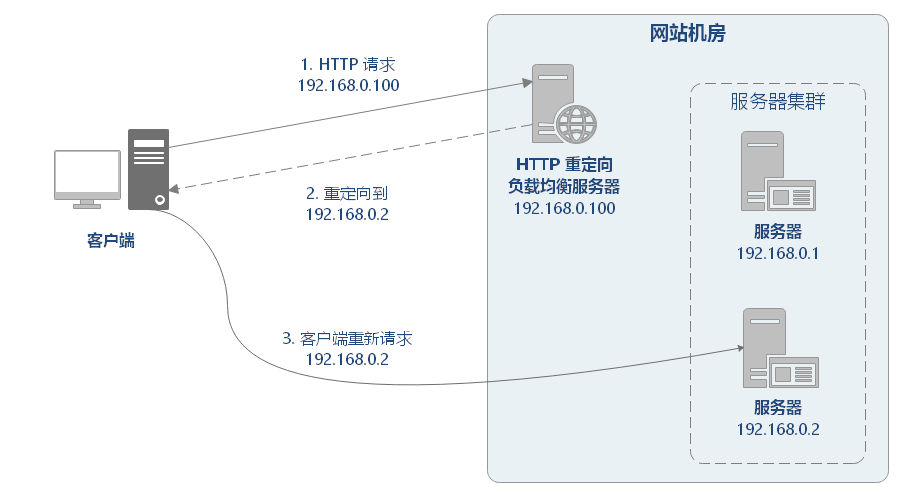HTTP 重定向的优点：方案简单。

HTTP 重定向的 缺点：

#### 2.2.3 反向代理负载均衡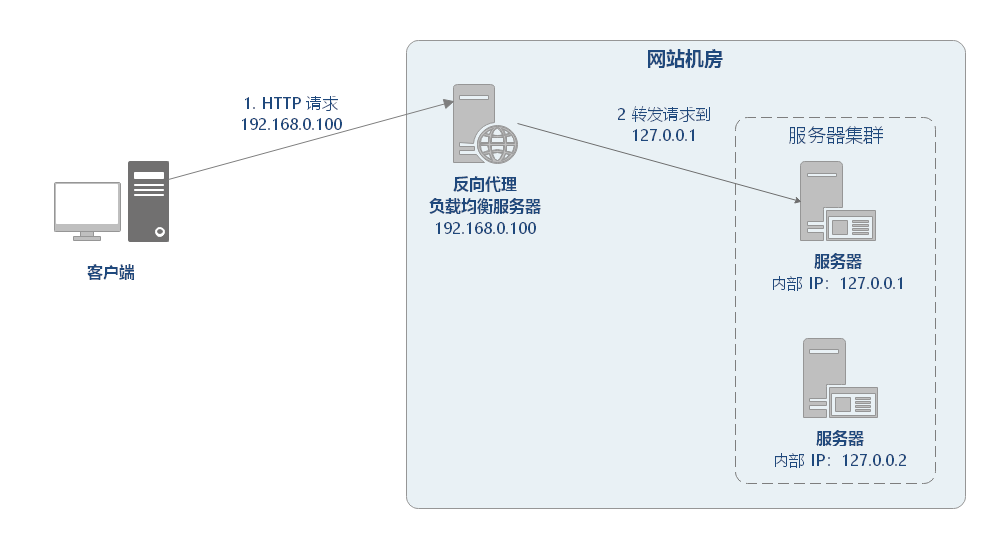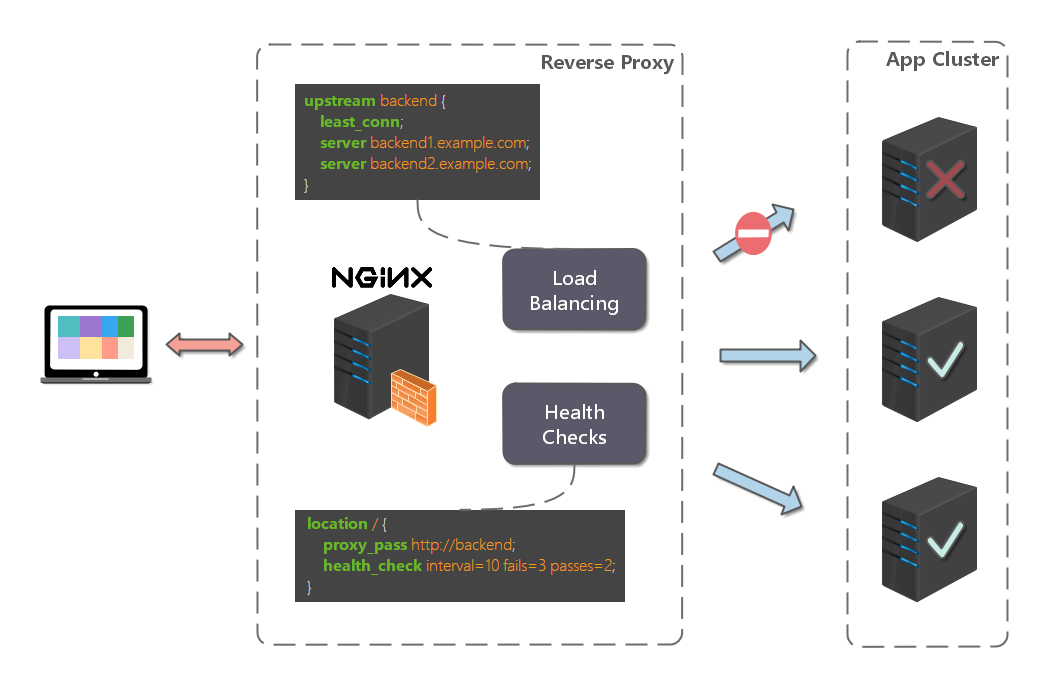1) 多种负载均衡算法：支持多种负载均衡算法，以应对不同的场景需求。

2) 可以监控服务器：基于 HTTP 协议，可以监控转发服务器的状态，如：系统负载、响应时间、是否可用、连接数、流量等，从而根据这些数据调整负载均衡的策略。

1) 额外的转发开销：反向代理的转发操作本身是有性能开销的，可能会包括创建连接，等待连接响应，分析响应结果等操作。

2) 增加系统复杂度：反向代理常用于做分布式应用的水平扩展，但反向代理服务存在以下问题，为了解决以下问题会给系统整体增加额外的复杂度和运维成本：

• 反向代理服务如果自身宕机，就无法访问站点，所以需要有 高可用 方案，常见的方案有：主备模式（一主一备）、双主模式（互为主备）。

反向代理服务自身也存在性能瓶颈，随着需要转发的请求量不断攀升，需要有 可扩展 方案。

#### 2.2.4 IP 负载均衡

IP 负载均衡是在网络层通过修改请求目的地址进行负载均衡。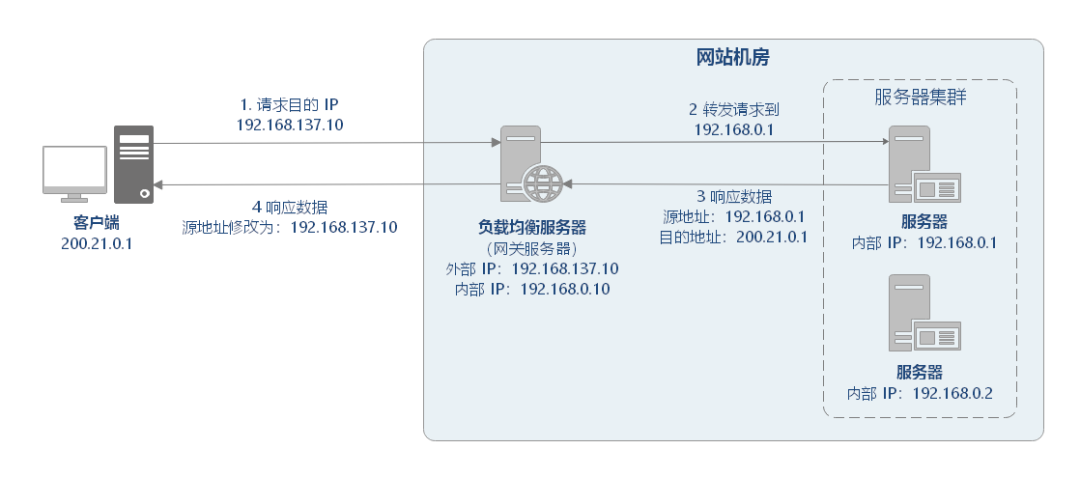IP 负载均衡在内核进程完成数据分发，较反向代理负载均衡有更好的从处理性能。但是，由于所有请求响应都要经过负载均衡服务器，集群的吞吐量受制于负载均衡服务器的带宽。

#### 2.2.5 数据链路层负载均衡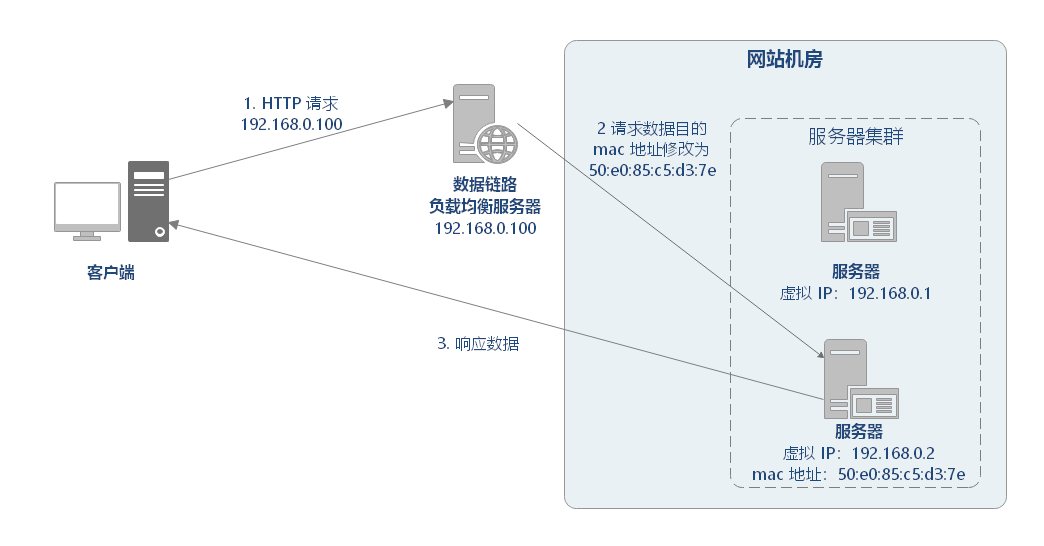LVS 的工作流程大致如下：

IPVS 是工作在 INPUT 链上，会根据访问的 vip+port 判断请求是否 IPVS 服务，如果是则调用注册的 IPVS HOOK 函数，进行 IPVS 相关主流程，强行修改数据包的相关数据，并将数据包发往 POSTROUTING 链上；

POSTROUTING 上收到数据包后，根据目标 IP 地址（后端服务器），通过路由选路，将数据包最终发往后端的服务器上。

## 三、负载均衡算法

### 3.1 随机

#### 3.1.1 随机算法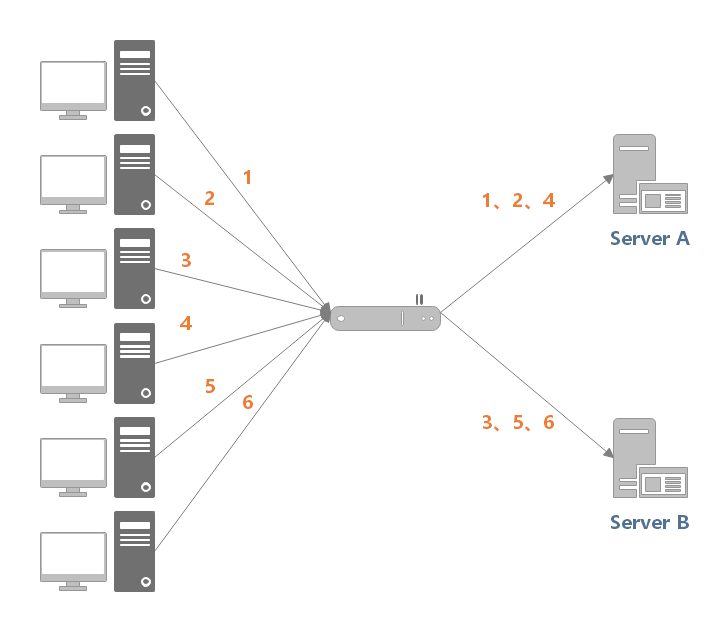【示例】随机算法实现示例

public interface LoadBalance<N extends Node> {    N select(List<N> nodes, String ip);}

public abstract class BaseLoadBalance<N extends Node> implements LoadBalance<N> {    @Override    public N select(List<N> nodes, String ip) {        if (CollectionUtil.isEmpty(nodes)) {            return null;        }        // 如果 nodes 列表中仅有一个 node，直接返回即可，无需进行负载均衡        if (nodes.size() == 1) {            return nodes.get(0);        }        return doSelect(nodes, ip);    }    protected abstract N doSelect(List<N> nodes, String ip);}

public class Node implements Comparable<Node> {    protected String url;    protected Integer weight;    protected Integer active;    // ...}

public class RandomLoadBalance<N extends Node> extends BaseLoadBalance<N> implements LoadBalance<N> {    private final Random random = new Random();    @Override    protected N doSelect(List<N> nodes, String ip) {        // 在列表中随机选取一个节点        int index = random.nextInt(nodes.size());        return nodes.get(index);    }}

#### 3.1.2 加权随机算法

【示例】加权随机算法实现示例

public class WeightRandomLoadBalance<N extends Node> extends BaseLoadBalance<N> implements LoadBalance<N> {    private final Random random = ThreadLocalRandom.current();    @Override    protected N doSelect(List<N> nodes, String ip) {        int length = nodes.size();        AtomicInteger totalWeight = new AtomicInteger(0);        for (N node : nodes) {            Integer weight = node.getWeight();            totalWeight.getAndAdd(weight);        }        if (totalWeight.get() > 0) {            int offset = random.nextInt(totalWeight.get());            for (N node : nodes) {                // 让随机值 offset 减去权重值                offset -= node.getWeight();                if (offset < 0) {                    // 返回相应的 Node                    return node;                }            }        }        // 直接随机返回一个        return nodes.get(random.nextInt(length));    }}

### 3.2 轮询

#### 3.2.1 轮询算法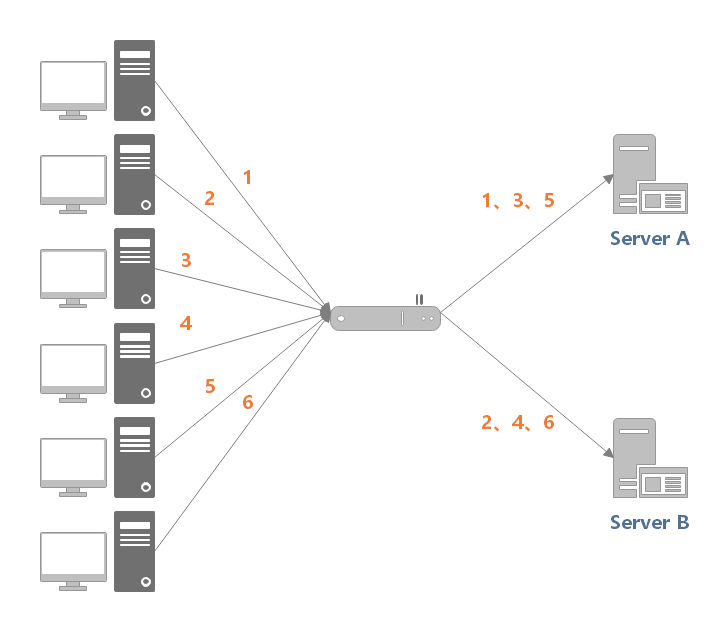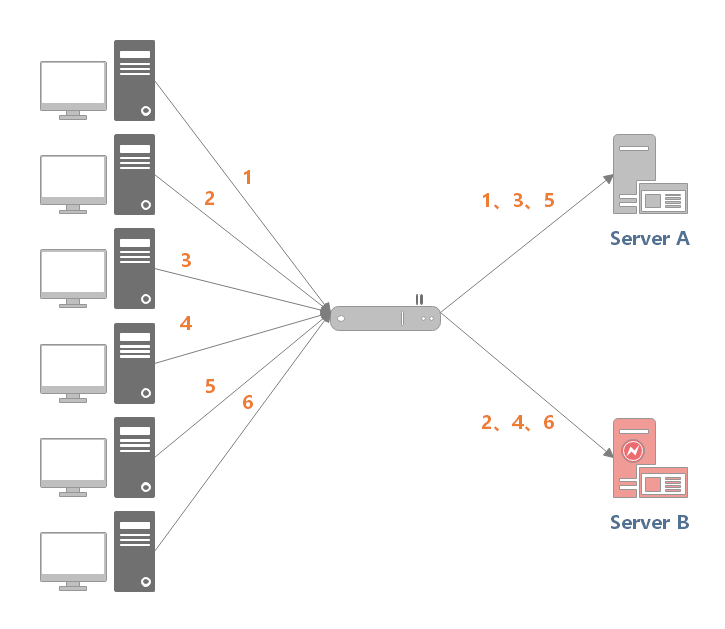【示例】轮询算法示例

public class RoundRobinLoadBalance<N extends Node> extends BaseLoadBalance<N> implements LoadBalance<N> {    private final AtomicInteger position = new AtomicInteger(0);    @Override    protected N doSelect(List<N> nodes, String ip) {        int length = nodes.size();        // 如果位置值已经等于节点数，重置为 0        position.compareAndSet(length, 0);        N node = nodes.get(position.get());        position.getAndIncrement();        return node;    }}

#### 3.2.2 加权轮询算法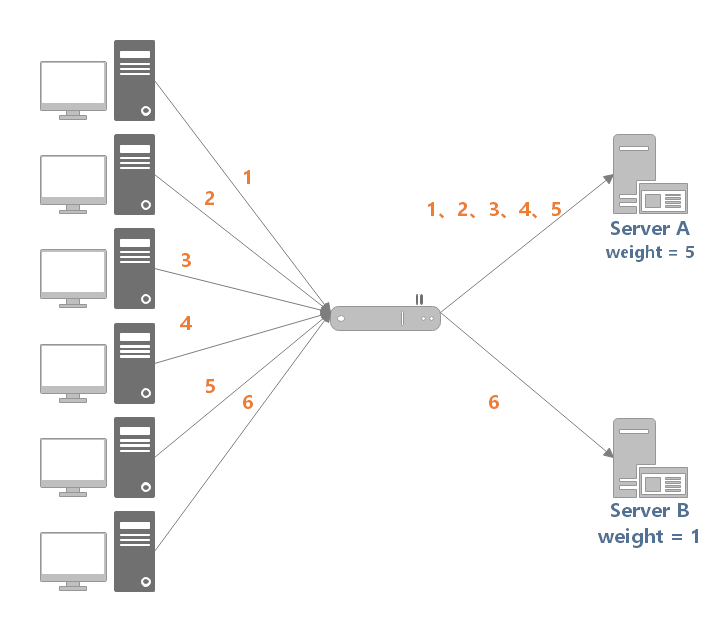【示例】加权轮询算法实现示例

public class WeightRoundRobinLoadBalance<N extends Node> extends BaseLoadBalance<N> implements LoadBalance<N> {    /**     * 60秒     */    private static final int RECYCLE_PERIOD = 60000;    /**     * Node hashcode 到 WeightedRoundRobin 的映射关系     */    private ConcurrentMap<Integer, WeightedRoundRobin> weightMap = new ConcurrentHashMap<>();    /**     * 原子更新锁     */    private AtomicBoolean updateLock = new AtomicBoolean();    @Override    protected N doSelect(List<N> nodes, String ip) {        int totalWeight = 0;        long maxCurrent = Long.MIN_VALUE;        // 获取当前时间        long now = System.currentTimeMillis();        N selectedNode = null;        WeightedRoundRobin selectedWRR = null;        // 下面这个循环主要做了这样几件事情：        //   1. 遍历 Node 列表，检测当前 Node 是否有相应的 WeightedRoundRobin，没有则创建        //   2. 检测 Node 权重是否发生了变化，若变化了，则更新 WeightedRoundRobin 的 weight 字段        //   3. 让 current 字段加上自身权重，等价于 current += weight        //   4. 设置 lastUpdate 字段，即 lastUpdate = now        //   5. 寻找具有最大 current 的 Node，以及 Node 对应的 WeightedRoundRobin，        //      暂存起来，留作后用        //   6. 计算权重总和        for (N node : nodes) {            int hashCode = node.hashCode();            WeightedRoundRobin weightedRoundRobin = weightMap.get(hashCode);            int weight = node.getWeight();            if (weight < 0) {                weight = 0;            }            // 检测当前 Node 是否有对应的 WeightedRoundRobin，没有则创建            if (weightedRoundRobin == null) {                weightedRoundRobin = new WeightedRoundRobin();                // 设置 Node 权重                weightedRoundRobin.setWeight(weight);                // 存储 url 唯一标识 identifyString 到 weightedRoundRobin 的映射关系                weightMap.putIfAbsent(hashCode, weightedRoundRobin);                weightedRoundRobin = weightMap.get(hashCode);            }            // Node 权重不等于 WeightedRoundRobin 中保存的权重，说明权重变化了，此时进行更新            if (weight != weightedRoundRobin.getWeight()) {                weightedRoundRobin.setWeight(weight);            }            // 让 current 加上自身权重，等价于 current += weight            long current = weightedRoundRobin.increaseCurrent();            // 设置 lastUpdate，表示近期更新过            weightedRoundRobin.setLastUpdate(now);            // 找出最大的 current            if (current > maxCurrent) {                maxCurrent = current;                // 将具有最大 current 权重的 Node 赋值给 selectedNode                selectedNode = node;                // 将 Node 对应的 weightedRoundRobin 赋值给 selectedWRR，留作后用                selectedWRR = weightedRoundRobin;            }            // 计算权重总和            totalWeight += weight;        }        // 对 weightMap 进行检查，过滤掉长时间未被更新的节点。        // 该节点可能挂了，nodes 中不包含该节点，所以该节点的 lastUpdate 长时间无法被更新。        // 若未更新时长超过阈值后，就会被移除掉，默认阈值为60秒。        if (!updateLock.get() && nodes.size() != weightMap.size()) {            if (updateLock.compareAndSet(false, true)) {                try {                    // 遍历修改，即移除过期记录                    weightMap.entrySet().removeIf(item -> now - item.getValue().getLastUpdate() > RECYCLE_PERIOD);                } finally {                    updateLock.set(false);                }            }        }        if (selectedNode != null) {            // 让 current 减去权重总和，等价于 current -= totalWeight            selectedWRR.decreaseCurrent(totalWeight);            // 返回具有最大 current 的 Node            return selectedNode;        }        // should not happen here        return nodes.get(0);    }    protected static class WeightedRoundRobin {        // 服务提供者权重        private int weight;        // 当前权重        private AtomicLong current = new AtomicLong(0);        // 最后一次更新时间        private long lastUpdate;        public long increaseCurrent() {            // current = current + weight；            return current.addAndGet(weight);        }        public long decreaseCurrent(int total) {            // current = current - total;            return current.addAndGet(-1 * total);        }        public int getWeight() {            return weight;        }        public void setWeight(int weight) {            this.weight = weight;            // 初始情况下，current = 0            current.set(0);        }        public AtomicLong getCurrent() {            return current;        }        public void setCurrent(AtomicLong current) {            this.current = current;        }        public long getLastUpdate() {            return lastUpdate;        }        public void setLastUpdate(long lastUpdate) {            this.lastUpdate = lastUpdate;        }    }}

### 3.3 最小活跃数

• 特点：根据候选服务器当前的请求连接数，动态分配。

• 场景：适用于对系统负载较为敏感或请求连接时长相差较大的场景。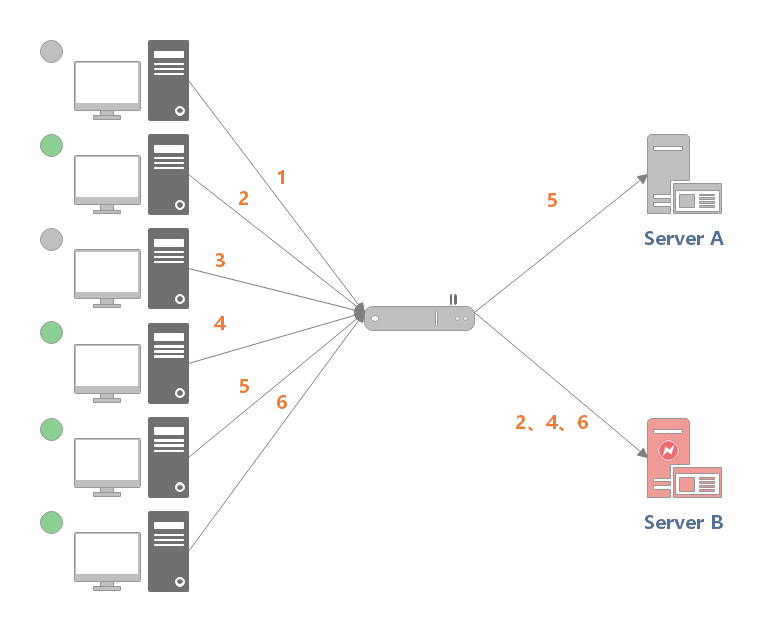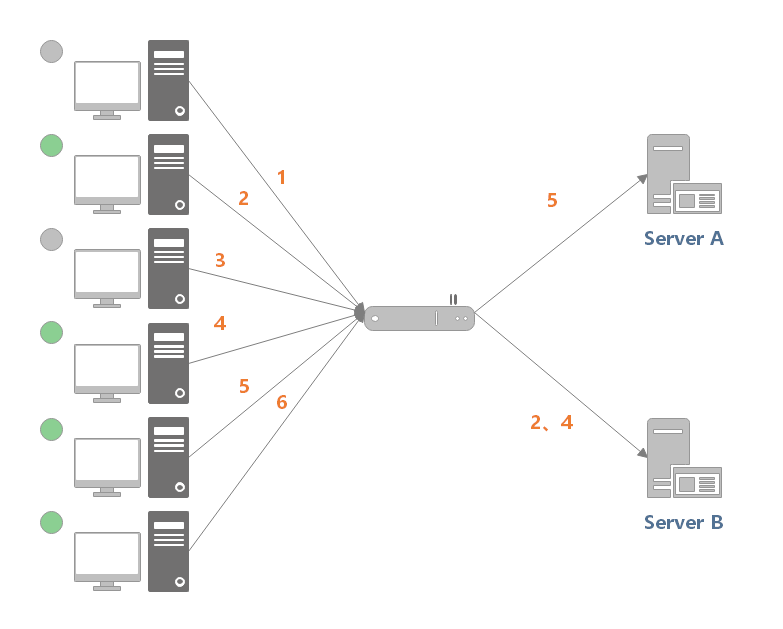【示例】最小活跃数算法实现

public class LeastActiveLoadBalance<N extends Node> extends BaseLoadBalance<N> implements LoadBalance<N> {    private final Random random = new Random();    @Override    protected N doSelect(List<N> nodes, String ip) {        int length = nodes.size();        // 最小的活跃数        int leastActive = -1;        // 具有相同“最小活跃数”的服务者提供者（以下用 Node 代称）数量        int leastCount = 0;        // leastIndexs 用于记录具有相同“最小活跃数”的 Node 在 nodes 列表中的下标信息        int[] leastIndexs = new int[length];        int totalWeight = 0;        // 第一个最小活跃数的 Node 权重值，用于与其他具有相同最小活跃数的 Node 的权重进行对比，        // 以检测是否“所有具有相同最小活跃数的 Node 的权重”均相等        int firstWeight = 0;        boolean sameWeight = true;        // 遍历 nodes 列表        for (int i = 0; i < length; i++) {            N node = nodes.get(i);            // 发现更小的活跃数，重新开始            if (leastActive == -1 || node.getActive() < leastActive) {                // 使用当前活跃数更新最小活跃数 leastActive                leastActive = node.getActive();                // 更新 leastCount 为 1                leastCount = 1;                // 记录当前下标值到 leastIndexs 中                leastIndexs = i;                totalWeight = node.getWeight();                firstWeight = node.getWeight();                sameWeight = true;                // 当前 Node 的活跃数 node.getActive() 与最小活跃数 leastActive 相同            } else if (node.getActive() == leastActive) {                // 在 leastIndexs 中记录下当前 Node 在 nodes 集合中的下标                leastIndexs[leastCount++] = i;                // 累加权重                totalWeight += node.getWeight();                // 检测当前 Node 的权重与 firstWeight 是否相等，                // 不相等则将 sameWeight 置为 false                if (sameWeight && i > 0                    && node.getWeight() != firstWeight) {                    sameWeight = false;                }            }        }        // 当只有一个 Node 具有最小活跃数，此时直接返回该 Node 即可        if (leastCount == 1) {            return nodes.get(leastIndexs);        }        // 有多个 Node 具有相同的最小活跃数，但它们之间的权重不同        if (!sameWeight && totalWeight > 0) {            // 随机生成一个 [0, totalWeight) 之间的数字            int offsetWeight = random.nextInt(totalWeight);            // 循环让随机数减去具有最小活跃数的 Node 的权重值，            // 当 offset 小于等于0时，返回相应的 Node            for (int i = 0; i < leastCount; i++) {                int leastIndex = leastIndexs[i];                // 获取权重值，并让随机数减去权重值                offsetWeight -= nodes.get(leastIndex).getWeight();                if (offsetWeight <= 0) {                    return nodes.get(leastIndex);                }            }        }        // 如果权重相同或权重为0时，随机返回一个 Node        return nodes.get(leastIndexs[random.nextInt(leastCount)]);    }}

### 3.4 源地址哈希

【示例】源地址哈希算法实现示例

public class IpHashLoadBalance<N extends Node> extends BaseLoadBalance<N> implements LoadBalance<N> {    @Override    protected N doSelect(List<N> nodes, String ip) {        if (StrUtil.isBlank(ip)) {            ip = "127.0.0.1";        }        int length = nodes.size();        int index = hash(ip) % length;        return nodes.get(index);    }    public int hash(String text) {        return HashUtil.fnvHash(text);    }}

### 3.5 一致性哈希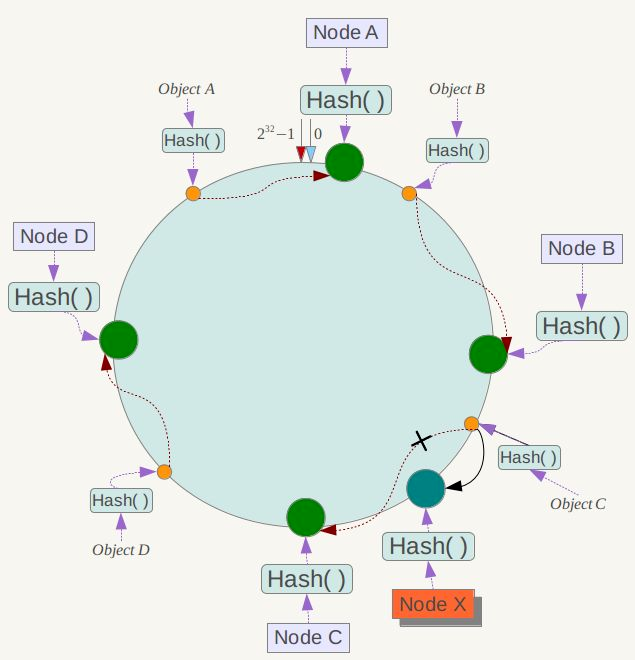1）相同的请求是指：一般在使用一致性哈希时，需要指定一个 key 用于 hash 计算，可能是：

2）尽可能是指：服务器可能发生上下线，少数服务器的变化不应该影响大多数的请求。

【示例】一致性哈希算法示例

public class ConsistentHashLoadBalance<N extends Node> extends BaseLoadBalance<N> implements LoadBalance<N> {    private final ConcurrentMap<String, ConsistentHashSelector<?>> selectors = new ConcurrentHashMap<>();    @SuppressWarnings("unchecked")    @Override    protected N doSelect(List<N> nodes, String ip) {        // 分片数，这里设为节点数的 4 倍        Integer replicaNum = nodes.size() * 4;        // 获取 nodes 原始的 hashcode        int identityHashCode = System.identityHashCode(nodes);        // 如果 nodes 是一个新的 List 对象，意味着节点数量发生了变化        // 此时 selector.identityHashCode != identityHashCode 条件成立        ConsistentHashSelector<N> selector = (ConsistentHashSelector<N>) selectors.get(ip);        if (selector == null || selector.identityHashCode != identityHashCode) {            // 创建新的 ConsistentHashSelector            selectors.put(ip, new ConsistentHashSelector<>(nodes, identityHashCode, replicaNum));            selector = (ConsistentHashSelector<N>) selectors.get(ip);        }        // 调用 ConsistentHashSelector 的 select 方法选择 Node        return selector.select(ip);    }    /**     * 一致性哈希选择器     */    private static final class ConsistentHashSelector<N extends Node> {        /**         * 存储虚拟节点         */        private final TreeMap<Long, N> virtualNodes;        private final int identityHashCode;        /**         * 构造器         *         * @param nodes            节点列表         * @param identityHashCode hashcode         * @param replicaNum       分片数         */        ConsistentHashSelector(List<N> nodes, int identityHashCode, Integer replicaNum) {            this.virtualNodes = new TreeMap<>();            this.identityHashCode = identityHashCode;            // 获取虚拟节点数，默认为 100            if (replicaNum == null) {                replicaNum = 100;            }            for (N node : nodes) {                for (int i = 0; i < replicaNum / 4; i++) {                    // 对 url 进行 md5 运算，得到一个长度为16的字节数组                    byte[] digest = md5(node.getUrl());                    // 对 digest 部分字节进行 4 次 hash 运算，得到四个不同的 long 型正整数                    for (int j = 0; j < 4; j++) {                        // h = 0 时，取 digest 中下标为 0 ~ 3 的4个字节进行位运算                        // h = 1 时，取 digest 中下标为 4 ~ 7 的4个字节进行位运算                        // h = 2, h = 3 时过程同上                        long m = hash(digest, j);                        // 将 hash 到 node 的映射关系存储到 virtualNodes 中，                        // virtualNodes 需要提供高效的查询操作，因此选用 TreeMap 作为存储结构                        virtualNodes.put(m, node);                    }                }            }        }        public N select(String key) {            // 对参数 key 进行 md5 运算            byte[] digest = md5(key);            // 取 digest 数组的前四个字节进行 hash 运算，再将 hash 值传给 selectForKey 方法，            // 寻找合适的 Node            return selectForKey(hash(digest, 0));        }        private N selectForKey(long hash) {            // 查找第一个大于或等于当前 hash 的节点            Map.Entry<Long, N> entry = virtualNodes.ceilingEntry(hash);            // 如果 hash 大于 Node 在哈希环上最大的位置，此时 entry = null，            // 需要将 TreeMap 的头节点赋值给 entry            if (entry == null) {                entry = virtualNodes.firstEntry();            }            // 返回 Node            return entry.getValue();        }    }    /**     * 计算 hash 值     */    public static long hash(byte[] digest, int number) {        return (((long) (digest[3 + number * 4] & 0xFF) << 24)            | ((long) (digest[2 + number * 4] & 0xFF) << 16)            | ((long) (digest[1 + number * 4] & 0xFF) << 8)            | (digest[number * 4] & 0xFF))            & 0xFFFFFFFFL;    }    /**     * 计算 MD5 值     */    public static byte[] md5(String value) {        MessageDigest md5;        try {            md5 = MessageDigest.getInstance("MD5");        } catch (NoSuchAlgorithmException e) {            throw new IllegalStateException(e.getMessage(), e);        }        md5.reset();        byte[] bytes = value.getBytes(StandardCharsets.UTF_8);        md5.update(bytes);        return md5.digest();    }}

## 四、参考资料

2. 《大型网站技术架构：核心原理与案例分析》

4. 什么是负载均衡## 评论